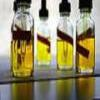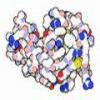#### You may also like### Mathematical Issues for Chemists

A brief outline of the mathematical issues faced by chemistry students.### Reaction Rates

Explore the possibilities for reaction rates versus concentrations with this non-linear differential equation### Mixed up Mixture

Can you fill in the mixed up numbers in this dilution calculation?

# Heavy Hydrocarbons

##### Age 14 to 18 Challenge Level:

Note that in actuality the masses of the different isotopologues of $\text{CH}_4$ are slightly different. These differences may be noted by a very sensitive mass spectrometer. Take for example:

RMM $^{12}\text{CH}_3\text{D}$ = 12 + 3(1.007825) + 2.014102 = 17.037577 gmol$^{-1}$
RMM $^{13}\text{CH}_4$ = 13.00335 + 4(1.007825) = 17.03465 gmol$^{-1}$
where the calculation is limited by the degree of accuracy of the given data.

However in this question it is acceptable to take values to the nearest gmol$^{-1}$, giving roughly equal molecular masses for certain isotopes.

$^{12}\text{CH}_4$ = 16 gmol$^{-1}$
$^{12}\text{CH}_3\text{D}$, $^{13}\text{CH}_4$ = 17 gmol$^{-1}$
$^{12}\text{CH}_2\text{D}_2$, $^{13}\text{CH}_3\text{D}$ = 18 gmol$^{-1}$
$^{12}\text{CHD}_3$, $^{13}\text{CH}_2\text{D}_2$ = 19 gmol$^{-1}$
$^{12}\text{CD}_4$, $^{13}\text{CHD}_3$ = 20 gmol$^{-1}$
$^{13}\text{CD}_4$ = 21 gmol$^{-1}$

The three most common species encountered would be $^{12}\text{CH}_4$, $^{13}\text{CH}_4$ and $^{12}\text{CH}_3\text{D}$ in order of likelihood. This can bee seen intuitively as the probability of encountering a $^{13}\text{C}$ more likely than encountering a single $^{2}\text{H}$, and for a small molecule such as methane, it is far more likely to obtain $^{12}\text{CH}_4$ than either of the other possibilities. The three most likely molecular masses as 16, 17 and 18 gmol$^{-1}$ as the introduction of more $^{13}\text{C}$ and $^{2}\text{H}$ to a small molecule reduces its likelihood more than any combinatorial effects can compensate for.

The actual probabilities of encountering each of these molecular masses of methane are:

16 gmol$^{-1}$ [$^{12}\text{CH}_4$]
$\textbf P(16 \text{gmol}^{-1}) = 0.989 \times (0.99985)^4 = 0.988$ (3sf)

17 gmol$^{-1}$ [$^{12}\text{CH}_3\text{D}$, $^{13}\text{CH}_4$]
$\textbf P(17 \text{gmol}^{-1}) = (0.989\times^4\textbf{C}_1(0.00015)\times (0.99985)^3 ) + (0.011 \times (0.99985)^4) = 0.0116$ (3sf)

18 gmol$^{-1}$ [$^{12}\text{CH}_2\text{D}_2$, $^{13}\text{CH}_3\text{D}$]
$\textbf P(18 \text{gmol}^{-1}) = (0.989\times^4\textbf{C}_2(0.00015)^2\times (0.99985)^2 ) + (0.011\times^4\textbf{C}_1(0.00015)\times (0.99985)^3) = 6.73\times10^{-6}$ (3sf)

19 gmol$^{-1}$ [$^{12}\text{CHD}_3$, $^{13}\text{CH}_2\text{D}_2$]
$\textbf P(19 \text{gmol}^{-1}) = (0.989\times^4\textbf{C}_3(0.00015)^3\times 0.99985 ) + (0.011\times^4\textbf{C}_2(0.00015)^2\times (0.99985)^2) = 1.50\times10^{-9}$ (3sf)

20 gmol$^{-1}$ [$^{12}\text{CD}_4$, $^{13}\text{CHD}_3$]
$\textbf P(20 \text{gmol}^{-1}) = (0.989\times^4\textbf{C}_4(0.00015)^4) + (0.011\times^4\textbf{C}_3(0.00015)^3\times 0.99985) = 1.49\times10^{-13}$ (3sf)

21 gmol$^{-1}$ [$^{13}\text{CD}_4$]
$\textbf P(21 \text{gmol}^{-1}) = (0.011\times^4\textbf{C}_4(0.00015)^4) = 5.57\times10^{-18}$ (3sf)

Following a similar principle to that above, the three most likely possibilities for the molecular masses of ethane are 30, 31 and 32 gmol$^{-1}$ in order of likelihood.

$^{12}\text{C}_2\text{H}_6$ = 30 gmol$^{-1}$
$^{12}\text{CD}_2\text{H}_5$, $^{12}\text{C}^{13}\text{C}\text{H}_6$ = 31 gmol$^{-1}$
$^{12}\text{C}_2\text{H}_4\text{D}_2$, $^{12}\text{C}^{13}\text{CD}\text{H}_5$, $^{13}\text{C}_2\text{H}_6$ = 32 gmol$^{-1}$

$\textbf P(30 \text{gmol}^{-1}) = (0.989)^2 \times (0.99985)^6 = 0.977$ (3sf)

$\textbf P(31 \text{gmol}^{-1}) = ((0.989)^2 \times ^6\textbf{C}_10.00015 \times (0.99985)^ 5) + ((0.989)\times^2\textbf{C}_1(0.011)\times(0.99985)^ 6) = 0.0226$ (3sf)

$\textbf P(32 \text{gmol}^{-1}) = ((0.989)^2 \times ^6\textbf{C}_2(0.00015)^2 \times (0.99985)^ 4) + ((0.989)\times^2\textbf{C}_1(0.011)\times^6\textbf{C}_1(0.00015)\times(0.99985)^ 5)$
$+ (^2\textbf{C}_2(0.011)^2 \times (0.99985)^6) = 1.41 \times 10^{-4} \text{ (3sf)}$

The three most likely possibilities for the molecular mass of propane are 44, 45 and 46 gmol$^{-1}$ in order of likelihood.

$^{12}\text{C}_3\text{H}_8$ = 44 gmol$^{-1}$
$^{12}\text{C}_2{}^{13}\text{C}\text{H}_8$, $^{12}\text{C}_3\text{D}\text{H}_7 = 45\text {gmol}^{-1}$
$^{12}\text{C}^{13}\text{C}_2\text{H}_8$, $^{12}\text{C}_2^{13}\text{C}\text{D}\text{H}_7, ^{12}\text{C}_3\text{D}_2\text{H}_ 6 = 46\text {gmol}^{-1}$

$\textbf P(44 \text{gmol}^{-1}) = (0.989)^3 \times (0.99985)^8 = 0.966$ (3sf)

$\textbf P(45 \text{gmol}^{-1}) = (^3\textbf{C}_1 \times 0.011 \times (0.989)^2 \times (0.99985)^ 8) + ((0.989)^3\times^8\textbf{C}_1(0.00015)\times(0.99985)^ 7) = 0.0334$ (3sf)

$\textbf P(46 \text{gmol}^{-1}) = (^3\textbf{C}_2 \times (0.011)^2 \times 0.989 \times (0.99985)^ 8) + (^3\textbf{C}_1 \times 0.011 \times (0.989)^2 \times^8\textbf{C}_1(0.00015)\times(0.99985)^ 7)$
$+ ((0.989)^3\times^8\textbf{C}_2(0.00015)^2\times(0.99985)^ 6) = 3.98 \times 10^{-4}$ (3sf)

A molecule of butane with molecular mass 72 is the isotopologue $^{13}\text{C}_4\text{D}_{10}$. The probability of any butane molecule being this isotopologue is:

$\textbf{P}(^{13}\text{C}_4\text{D}_{10}) = 0.011^4 \times 0.00015^{10} = 8.44 \times 10^{-47}$ (3sf)

24dm$^3$ of butane corresponds to roughly 1 mole of butane molecules. Thus, as 1 dm$^3$ is equivalent to a litre, the number of moles in the required volume is $\frac{1}{24}$.

The number of molecules in the sample is given by multiplying the number of moles, by the number of molecules in a mole (the Avogadro's constant):

Number of molecules $= \frac{N_A}{24} = 2.51 \times 10^{22}$

So the likelihood can be found by multiplying the probability for one molecule by the total number of molecules in the sample.

$\therefore\text{E}(^{13}\text{C}_4\text{D}_{10}) = 2.51 \times 10^{22} \times 8.44 \times 10^{-47} = 2.12 \times 10^{-24}$

This question requires a little more algebraic appreciation of the calculations thusfar.
The probability of encountering a generic alkane $^{12}\text{C}_n{}^1\text{H}_{2n + 2}$ is given by:

$\textbf{P} = 0.989^n \times (0.99985)^{2n + 2}$

The probability of encountering an isotopologue containing deuterium $^{12}\text{C}_n^{\ 1}\text{H}_{2n + 1}\text{D}$ is given by:

$\textbf{P} = 0.989^n \times ^{2n + 2}\textbf{C}_1(0.99985)^{2n + 1}\times 0.00015$

The probability of encountering this isotopologue must be greater than the likelihood of finding the butane molecule consisting entirely of $^{12}\text{C}$ and H.

$0.989^n \times ^{2n + 2}\textbf{C}_1 \times (0.99985)^{2n + 1}\times 0.00015$ > $0.989^n \times (0.99985)^{2n + 2}$

$0.00015 (2n + 2)$ > 0.99985

2n + 2 > 6665.666667

n > 3331.833333

n = 3332

Is this long chain alkane likely to exist in a real sample?

The probability of encountering an isotopologue containing $^{13}\text{C}$ is given by:

$\textbf{P} = ^n\textbf{C}_1\times 0.011 \times 0.989^{n-1} \times (0.99985)^{2n + 2}$

This probability must be greater than the likelihood of finding the alkane molecule consisting entirely of $^{12}\text{C}$ and H. So:

$^n\textbf{C}_1\times 0.011 \times (0.989)^{n-1} \times (0.99985)^{2n + 2}$ > $0.989^n \times (0.99985)^{2n + 2}$

$\text{n} \times (0.989)^{n-1} \times 0.011$ > $(0.989)^{n}$

$0.011\text{n}$ > 0.989

$\text{n}$ > 89.90909091

n = 90

Consider the likelihood of the existence of this molecule.

As an extension, does the probability of the existence of such molecules change if the molecule is produced via a method which involves polymerisation? Try to construct an algebraic test.